Viscoelasticity Notes adapted from
Viscoelastic Materials
Rod Lakes
University of Wisconsin
Viscoelasticity main     Demonstrations     Nonlinearity     Class     Viscoelasticity Books '98,     '09     Biomechanics     Ultrasonics

Rod Lakes Home

§1.1 Viscoelastic phenomena. Internal friction.
Most structural engineering materials are described, for small strains, by Hooke's law of linear elasticity: stress (usually represented as a lower case Greek sigma) s is proportional to strain e. Strain is usually represented as a lower case Greek epsilon. If your web browser does not interpret Symbol font properly, e may instead look like a bold face e, and Greek pi may look like Latin p.
For an alternative page go here.
In one dimension, Hooke's law is as follows.
s = E e,        (1.1.1)
with E as Young's modulus. Hooke's law for elastic materials can also be written in terms of a compliance J:
e = J s.        (1.1.2)
Consequently the elastic compliance J is the inverse of the modulus E,
J = 1/E .        (1.1.3)
By contrast to elastic materials, a viscous fluid under shear stress obeys s = hde/dt, with h as the viscosity.

In reality all materials deviate from Hooke's law in various ways, for example by exhibiting viscous-like as well as elastic characteristics. Viscoelastic materials are those for which the relationship between stress and strain depends on time. Anelastic solids represent a subset of viscoelastic materials: they have a unique equilibrium configuration and ultimately recover fully after removal of a transient load.
Some phenomena in viscoelastic materials are: (i) if the stress is held constant, the strain increases with time (viscoelastic creep); (ii) if the strain is held constant,the stress decreases with time (viscoelastic relaxation); (iii) the effective stiffness depends on the rate of application of the load; (iv) if cyclic loading is applied, hysteresis (a phase lag) occurs, leading to a dissipation of mechanical energy; (v) acoustic waves experience attenuation; (vi) rebound of an object following an impact is less than 100%; (vii) during rolling,frictional resistance occurs.
All materials exhibit some viscoelastic response. In common metals such as steel or aluminum, as well as in quartz, at room temperature and at small strain, the behavior does not deviate much from linear elasticity. Synthetic polymers, wood, and human tissue as well as metals at high temperature display significant viscoelastic effects. In some applications, even a small viscoelastic response can be significant. To be complete, an analysis or design involving such materials must incorporate their viscoelastic behavior.
Knowledge of the viscoelastic response of a material is based on measurement. The mathematical formulation of viscoelasticity theory is presented in the following chapters with the aim of enabling prediction of the material response to arbitrary load histories.

§ 1.2 Motivations for studying viscoelasticity
The study of viscoelastic behavior in bone and in bio-protective materials is of interest in several contexts. Materials used for structural applications of practical interest may exhibit viscoelastic behavior which has a profound influence on the performance of that material. For example, viscoelastic shoe insoles are useful in reducing mechanical shocks transmitted to the bones and joints. Materials used in engineering applications may exhibit viscoelastic behavior as an unintentional side effect. In applications, one may deliberately make use of the viscoelasticity of certain materials in the design process, to achieve a particular goal. Viscoelasticity is of interest in materials science, metallurgy, and solid state physics since it is causally linked to a variety of microphysical processes and can be used as an experimental probe of those processes. The causal links between viscoelasticity and microstructure are exploited in the use of viscoelastic tests as an inspection or diagnostic tool.

§1.3 Transient properties: creep and relaxation
§1.3.1 Viscoelastic functions
Creep is a slow, progressive deformation of a material under constant stress. In one dimension, suppose the history of stress s as it depends on time t to be a step function beginning at time zero:

s(t) = s0H(t).        (1.3.1)

H(t) is the unit Heaviside step function defined as zero for t less than zero, one for t greater than zero, and 1/2 for t = 0. The strain e(t) in a viscoelastic material will increase with time. The ratio

J(t) = ( e(t) / s0)        (1.3.2)

is called the creep compliance. In linearly viscoelastic materials, the creep compliance is independent of stress level. The intercept of the creep curve on the strain axis is ascribed by some authors to "instantaneous elasticity". However no load can be physically applied instantaneously. If the loading curve is viewed as a mathematical step function, we remark that the region around zero time contains an infinite domain on a logarithmic scale, a topic we shall return to later. If the load is released at a later time, the strain will exhibit recovery, or progressive decrease of deformation. Strain in recovery may or may not approach zero, depending on the material. We remark that the recovery phase is not included in Eq. 1.3.1 and 1.3.2.
The following demonstration illustrates the logarithmic nature of creep. Images are taken at logarithmic intervals, 1 second, 10 seconds, 100 seconds, and so on, in the animation.
Viscoelasticity Demonstration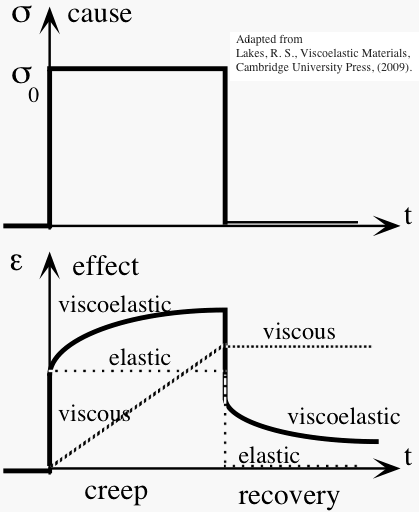Fig. 1.1 Creep and recovery. Stress s and strain e vs. time t.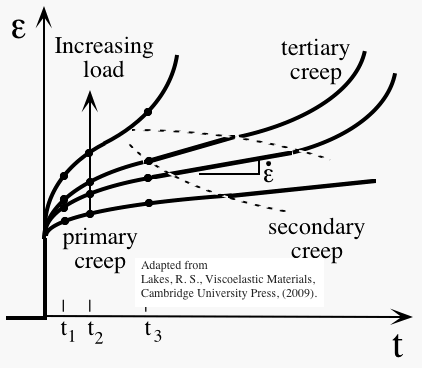Fig. 1.2 Regions of creep behavior. Strain e vs. time t, for different load levels.

Elastic materials constitute a special case for which the creep compliance is
J(t) = J 0 H(t),
with J 0 as a constant which is the elastic compliance. Elastic materials exhibit immediate "recovery" to zero strain following release of the load. Viscoelastic materials which exhibit complete recovery after sufficient time following creep or relaxation are called anelastic. Viscous materials constitute another special case in which the creep compliance is
J(t) = h t H(t),
with h as the viscosity. Creep deformation in viscous materials is unbounded.
The creep response in Fig. 1.1 is shown beginning at the same time as the stress history, which is the cause. The corresponding functional form is J(t) = j(t) H(t), with j(t) as a function defined over the entire time scale. This functional form for J(t) follows from the physical concept of causality, that the effect does not precede the cause.
Creep curves may exhibit three regions (Fig. 1.2), primary creep in which the curve is concave down (when plotted on a linear, not logarithmic time scale), secondary creep in which deformation is proportional to time, and tertiary creep in which deformation accelerates until creep rupture occurs. Tertiary creep is always a manifestation of nonlinear viscoelasticity, and secondary creep is usually nonlinear as well. Although secondary creep is represented by a straight line in a plot of strain vs. time, that straight line has nothing whatever to do with linear viscoelasticity. Linear response involves a linear relationship between cause and effect: stress and strain at a given time in the case of creep. Specifically, data taken at different load levels may be compared by considering isochronals or data at the same time. Data points at times t 1, t 2, and t 3 are illustrated in Fig. 1.2..
Stress relaxation is the gradual decrease of stress when the material is held at constant strain. If we suppose the strain history to be a step function beginning at time zero:
e(t) = e0H(t),
the stress s(t) in a viscoelastic material will decrease as shown in Figure 1.3.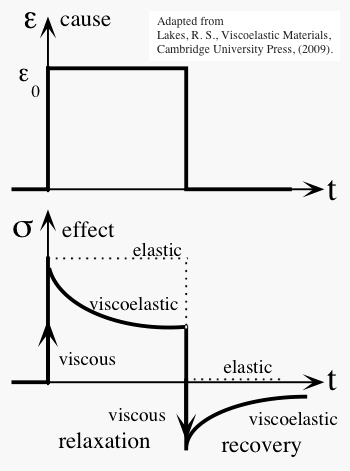The ratio

E(t) = ( s(t) / e0)        (1.3.3)

is called the relaxation modulus. In linear materials, it is independent of strain level, so E(t) is a function of time alone.
The symbol E for stiffness in uniaxial tension and compression is used in subsequent sections since the introductory presentations are restricted to one dimension. Creep and relaxation can occur in shear or in volumetric deformation as well. The relaxation function for shear is called G(t). For volumetric deformation, the elastic bulk modulus is called B (also called K). A corresponding relaxation function B(t) may be defined as above, but with the stress as a hydrostatic stress. A distinction is made in the creep compliances, J G(t) for creep in shear, J E(t) for creep in extension, and J B(t) for creep in volumetric deformation.

§1.4 Dynamic response to sinusoidal load
If a stress s(t) varying sinusoidally (Fig. 1.4) in time t,

s(t) = s0sin(2pnt)        (1.4.1)

of frequency n (in cycles per second or Hertz, abbreviated Hz) is applied to a linearly viscoelastic material, the strain

e(t) = e0sin(2pnt - d)        (1.4.2)

will also be sinusoidal in time but will lag the stress by a phase angle d. The period T of the waveform is the time required for one cycle: T = 1/ n.
The phase angle is related to the time lag Dt between the sinusoids by d = 2p( Dt)/T. To see this, the argument in Eq. 1.4.2 may be written
2pnt - d = 2p nt - (2p nd / 2pn) = 2p n(t - ( d / 2pn)) = 2p n(t - Dt).        (1.4.3)
So Dt = ( d / 2pn),        (1.4.4)
With n = (1/T) ,
d = (2p D t/T).        (1.4.5)
As a result of the phase lag between stress and strain, the dynamic stiffness can be treated as a complex number:
(s / e0) = E' + iE".        (1.4.6)
The single and double primes designate the real and imaginary parts; they do not represent derivatives. The loss angle d is a dimensionless measure of the viscoelastic damping of the material. The dynamic functions E', E", and d depend on frequency. The tangent of the loss angle is called the loss tangent: tan d. In an elastic solid, tan d = 0. The relationship between the transient properties E(t) and J(t) and the dynamic properties E', E" and tan d is developed in §3.2. Dynamic viscoelastic behavior, in particular tan d and its consequences, is at times referred to as internal friction or as mechanical damping .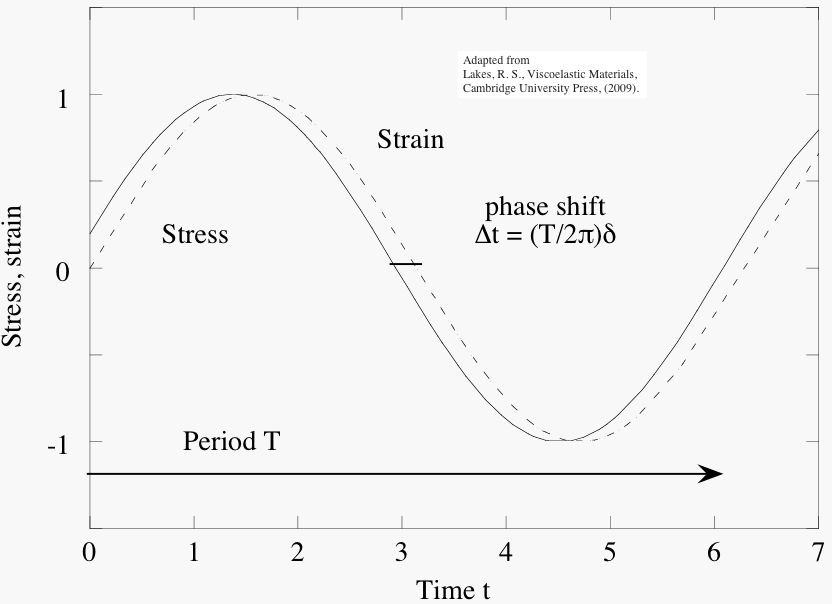Fig.1.4 Stress and strain vs. time t (in arbitrary units) in dynamic loading of a viscoelastic material.

§2 Dynamic properties: damping.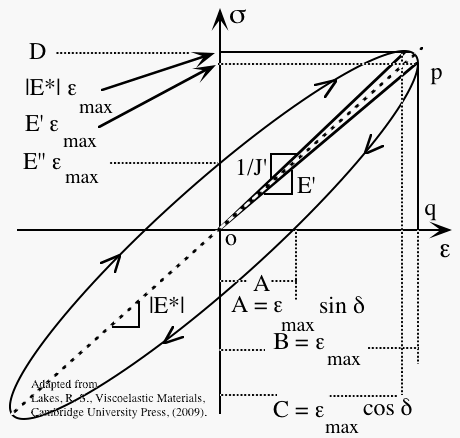Click on image for a bigger image.
This diagram shows a stress-strain curve for a linearly viscoelastic material. The role of the phase angle or loss angle d (delta) is illustrated.
The loss angle d, or the loss tangent tan d, may be considered as the fundamental measure of damping in a linear material. Other measures, such as those developed above, are often cited in analyses of viscoelastic behavior. For the purpose of comparison, these measures of damping and their relationship to the loss angle are presented in the following table.

Table 1. Measures of damping

Measure, Relation to d, Phenomenon
Loss angle d Phase, stress, strain
Loss tangent tan d Tangent of the phase angle
(E''(n) / E'(n)) = tan d(n) Ratio of imaginary part to real part of modulus
Quality factor Q = (tan d)-1..... Resonance peak width
Log decrement L =ptan d..... Free decay of vibration
Decay time t 1/e........ tan d = (1/p) (T/t 1/e)..... Free decay of vibration
Vibration goes on forever if there is no damping. Free decay of vibration is illustrated in the following demonstration. Strike a tuning fork. If the fork is made of aluminum, vibration continues for thousands of cycles, as you can hear from the tone, and visualize from the recorded waveform. Compare with forks made of wood, and different polymers in the following demonstration.
Viscoelasticity Demonstration

Specific Damping Capacity
L = 2ptan d Ratio of energy dissipated to energy stored
Attenuation al = 2p tan ( d / 2) Wave attenuation, (neper/ l)
Attenuation a = ( l/c) tan ( d/2) Wave attenuation, (neper/m)
Rod Lakes Home
Top